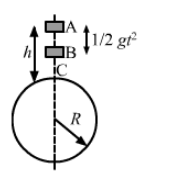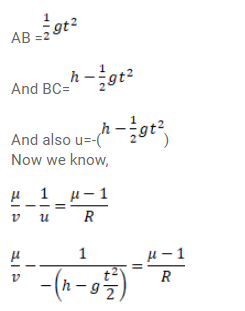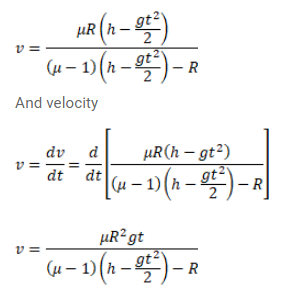Deepak Scored 45->99%ile with Bounce Back Crack Course. You can do it too!

# A ball is kept at a height h above the surface of a heavy

Question:

A ball is kept at a height $h$ above the surface of a heavy transparent sphere made of a material of refractive index $\mu$. The radius of the sphere is $\mathrm{R}$. At $\mathrm{t}=0$, the ball is dropped to fall normally on the sphere.

Find the speed of the image formed as a function of time for $t<\sqrt{\frac{2 h}{g}}$. Consider only the image by a single refraction.

Solution: